# Asset Beta & Market Beta In PythonBy Jay Maniar

In this blog, we will highlight the importance of understanding ‘beta’ in the stock market and how we can use beta effectively to hedge against market risk. We will even see how to calculate beta of any stock in python. So let us begin, by understanding a few basic questions that should come to our mind before we start coding in python.

### What is ‘beta’?

The volatility that a benchmark portfolio (S&P 500 index) or a market portfolio exhibits is known as systematic risk. Beta is the historical measure of risk of any individual stock or portfolio against the risk of the market portfolio. In other words, it measures the volatility of any security with respect to the overall market volatility. Let us consider the following example to understand beta intuitively.

Similarly, if the beta of any stock is say 0.75, then it will be less volatile than the market. If the intraday gains of the market are 10%, a low beta stock will gain only 7.5%. Low beta stocks are very useful to mitigate market risk. This is because, if the market declines by 5%, then the stock will decline by only 3.75%. This is less than the benchmark decline as opposed to the decline by Google when the market fell by 5%.

To begin, let us import the data in python and plot the daily returns of Google and S&P 500 index.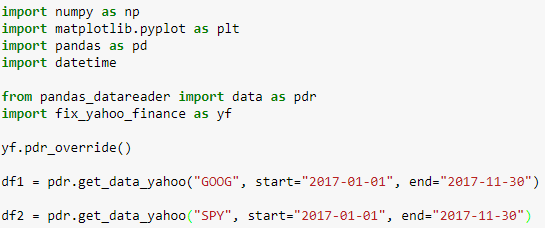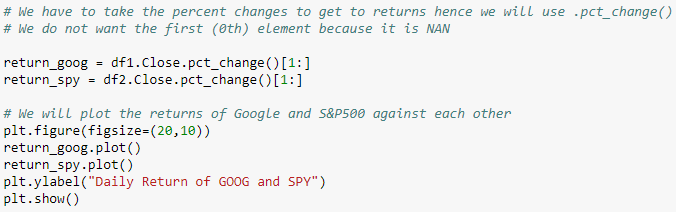Output: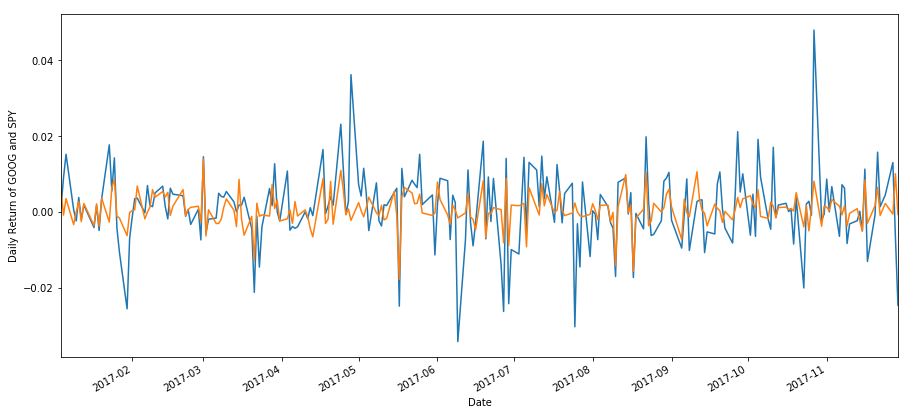The returns that are more volatile (in blue) are that of Google and the less volatile returns (in orange) are that of S&P 500 index.

### How is beta calculated?

One of the most common ways to calculate beta is using the Capital Asset Pricing Model or CAPM.

Have a look at the CAPM model:

`E (Ra) = Rf + Ba [ E (Rm) - Rf]`

CAPM model states that the expected return of an asset ‘E (Ra)’ is equal to the risk-free return in the market plus the difference between the expected return of the market and risk-free rate ‘[E (Rm) - Rf]’ multiplied by the asset’s beta  ‘Ba’.

If we have all the values except the asset’s beta, we can calculate the beta using:

` Ba = [E (Ra) - Rf] / [ E (Rm) - Rf]`

We can even find beta by performing the ‘regression analysis’. When one tries to capture a mathematical relationship between ‘x’ and ‘y’ variables, by fitting a line, polynomial or a curve through scatter plots, such that one can make a reasonably good prediction of  ‘y’ given ‘x’, then the mathematical process of deriving such an equation between x and y is called the regression analysis.This equation can also arrived at by using a machine learning based regression model.

If we try to fit a ‘line’ through this scatter plot that “best” explains the observed values of ‘y’ in terms of observed values of ‘x’, we get a simple linear regression model.

Linear regression assumes a linear relationship between the dependent and independent variables. The following regression equation describes that relation:

`Yi = b0 + b1 Xi + ei`

We refer to the intercept ‘b0’ and slope coefficient ‘b1’ as the regression coefficients and ei  as the random error.

Now, consider this equation:

`Rasset = ex-post alpha + beta of asset *  Rbenchmark  + ei`

To understand the coefficients more intuitively, if we consider the returns for Google vs. S&P 500 index, then the slope coefficient in a regression line is called the stock’s beta, as it measures the relative amount of systematic or undiversifiable risk in Google’s returns. If the slope of Google returns is more than 1, its returns tend to increase or decrease more than the market returns. A slope or beta of 1 would have the same level of systematic risk as that of the market on an average, and a slope or beta less than 1 implies that the returns increase or decrease by less than the change in the market returns.

The intercept term is the ex-post alpha i.e. the measure of excess returns of Google as compared to market index returns. If the intercept term is negative, it means Google has underperformed S&P on a risk adjusted basis and a positive intercept means it has had excess returns on risk adjusted basis.

All the points on the regression equation line, predict the ‘y’ values for the corresponding ‘x’ values. However, the optimal regression line is the one for which the sum of the squared differences (vertical distances) or the sum of squared errors or SSE between the ‘y’ values predicted by the regression equation/line and the actual ‘y’ values is minimal.

Thus, the regression line minimizes the SSE. This is the reason why simple linear regression is also called as Ordinary Least Squares or OLS, and the estimated (predicted) values by the regression equation i.e. y predicted are called least squares estimates.

The slope coefficient ‘b1’ of the regression line is calculated as the covariance of x and y divided by the variance of x (covxy 2x ), and the intercept coefficient is the line's intersection with the y axis at x = 0.

Consider the following code to calculate Google’s beta against S&P 500.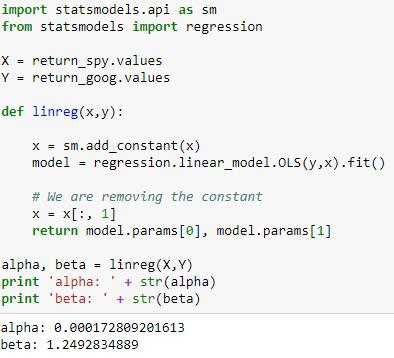We have calculated the beta of Google as 1.25. You may try this with other stocks.

Below is the linear regression plot.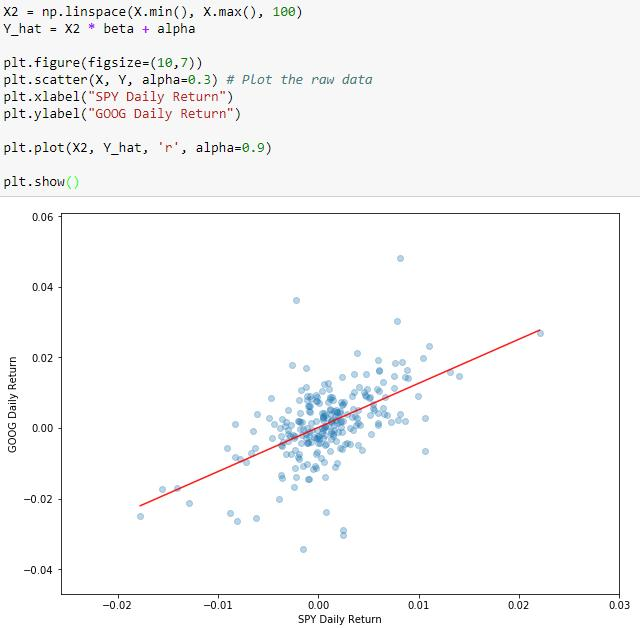This is where we will end this blog. We can even hedge the Google's beta with respect to the S&P 500 beta. This is known as beta hedging. We will cover this in the next part of the blog.

### Next Step

Learn about the Put-call parity concept in Python. It is a concept that describes how the value of call option, put option and the stock are inter-related. We cover this and much more in this blog.

Update

We have noticed that some users are facing challenges while downloading the market data from Yahoo and Google Finance platforms. In case you are looking for an alternative source for market data, you can use Quandl for the same.